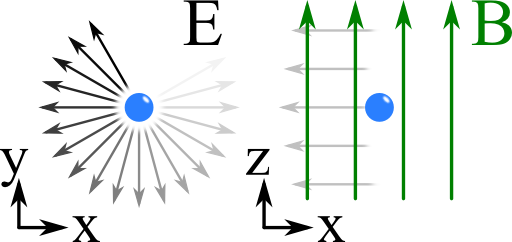## The Dispersion Relation of a Magnetized PlasmaIt is known that a free electron gas prone to a periodic electric field obeys oscillations. One might ask what happens if an additional external magnetic field is acting on the plasma. In this problem we will discover how the magnetic field changes the dispersion relation. This will later allow us to understand how the magnetic field in the universe is measured using the "Faraday Rotation".

## Problem StatementWe want to assume an electron in a constant magnetic field parallel to the $$z$$ axis, $$\mathbf{B}=B_{0}\mathbf{e}_{z}$$. The free (non-interacting) electrons shall be driven by a left/right circularly polarized plane wave propagating in $$z$$-direction, see figure on the right:

Find the equations of motion for the electron. Use the assumptions that the range of the electron movement is much smaller than the wavelength and that its speed is much slower than the speed of light. Solve the equations of motion to find the electron displacement. Then, calculate the dispersion relation of the system assuming an electron density $$n_{e}$$.

This problem is very helpful to understand "The Faraday Rotation - How an Electron Gas and a Magnetic Field Rotate a Plane Wave" and a generalization of the Drude model which describes the electrodynamic properties of metals.